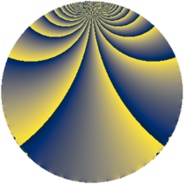# Properties

 Label 273.2.bzLevel $273$ Weight $2$ Character orbit 273.bz Rep. character $\chi_{273}(31,\cdot)$ Character field $\Q(\zeta_{12})$ Dimension $72$ Newform subspaces $2$ Sturm bound $74$ Trace bound $7$

# Related objects

## Defining parameters

 Level: $$N$$ $$=$$ $$273 = 3 \cdot 7 \cdot 13$$ Weight: $$k$$ $$=$$ $$2$$ Character orbit: $$[\chi]$$ $$=$$ 273.bz (of order $$12$$ and degree $$4$$) Character conductor: $$\operatorname{cond}(\chi)$$ $$=$$ $$91$$ Character field: $$\Q(\zeta_{12})$$ Newform subspaces: $$2$$ Sturm bound: $$74$$ Trace bound: $$7$$ Distinguishing $$T_p$$: $$2$$

## Dimensions

The following table gives the dimensions of various subspaces of $$M_{2}(273, [\chi])$$.

Total New Old
Modular forms 168 72 96
Cusp forms 136 72 64
Eisenstein series 32 0 32

## Trace form

 $$72q - 2q^{7} + 36q^{9} + O(q^{10})$$ $$72q - 2q^{7} + 36q^{9} + 8q^{11} - 72q^{14} + 24q^{16} - 30q^{19} + 8q^{21} - 16q^{22} - 36q^{24} - 60q^{26} + 16q^{28} - 32q^{29} - 6q^{31} + 20q^{32} + 16q^{35} + 14q^{37} + 2q^{39} + 120q^{40} + 24q^{42} + 8q^{44} - 16q^{46} - 136q^{50} - 144q^{52} - 16q^{53} - 12q^{57} - 48q^{58} + 96q^{59} - 44q^{60} + 72q^{61} + 2q^{63} - 16q^{65} - 2q^{67} + 72q^{68} - 104q^{70} - 72q^{71} - 54q^{73} + 80q^{74} + 64q^{78} - 12q^{80} - 36q^{81} + 20q^{84} + 88q^{85} + 24q^{86} + 72q^{87} + 48q^{89} - 10q^{91} - 80q^{92} + 6q^{93} + 132q^{96} + 96q^{98} + 16q^{99} + O(q^{100})$$

## Decomposition of $$S_{2}^{\mathrm{new}}(273, [\chi])$$ into newform subspaces

Label Dim. $$A$$ Field CM Traces $q$-expansion
$$a_2$$ $$a_3$$ $$a_5$$ $$a_7$$
273.2.bz.a $$36$$ $$2.180$$ None $$0$$ $$0$$ $$0$$ $$-6$$
273.2.bz.b $$36$$ $$2.180$$ None $$0$$ $$0$$ $$0$$ $$4$$

## Decomposition of $$S_{2}^{\mathrm{old}}(273, [\chi])$$ into lower level spaces

$$S_{2}^{\mathrm{old}}(273, [\chi]) \cong$$ $$S_{2}^{\mathrm{new}}(91, [\chi])$$$$^{\oplus 2}$$